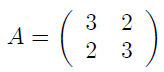# Solution Exercise 552 : python numpy code computing the reverse of given matrix A

#### Exercise 552

For Given Matrix A:1.  Give the python numpy code that compute the reverse B of matrix A
2. To ensure the result, calculate B.dot (A)

### Question 1

``import numpy as np A = np.array([[3,2] ,             [2,3]])B = np.linalg.inv (A)print ("The reverse of A is :", B)# The out put is : The reverse of A is : [[ 0.6 -0.4] [-0.4  0.6]]``

### Question 2

``import numpy as np A = np.array([[3,2] ,             [2,3]])B = np.linalg.inv(A)BA = np.dot(A,B)print(AB)The output is : [[ 1.00000000e+00  0.00000000e+00]  [-2.22044605e-16  1.00000000e+00]]``